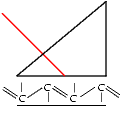# Simulation of the Reflectivity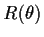A suitable theoretical method to describe the reflectivity and within the leaky modes of a thin layer or a multilayer system (see Figure below) is a matrix formalism after Yeh ["Optical Waves in layered media",John Wiley and Sons 1988]. The electromagnetic wave is decomposed into a left and a right propagating component.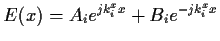(1)

The relation between the different Amplitudes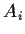and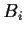is given by a matrix multiplication over all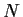layers.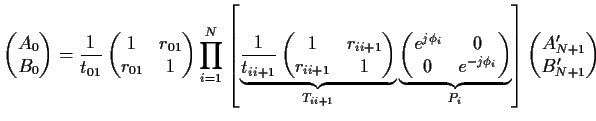(2)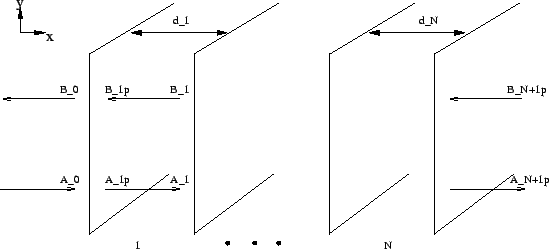From which the reflected intensity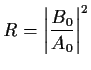(3)

can be calulated. The elements of the so called transfer Matrixare the fresnel coefficients for transmission and reflection at the interface between two different media. The optical phase difference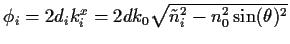is introduced by a phase matrix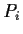. The complex refractive index leads to a real exponentional factor in the elements of the phase matrix which influences strongly the intensity of the reflected light.
This matrix calculation is a very general tool and it can easily be implemented on a computer calculating a large number (practically continuous) of layers N, simulating static or dynamic refractive index profiles.